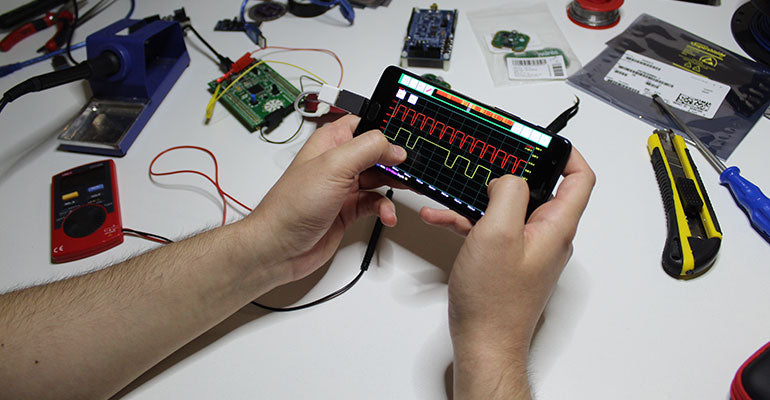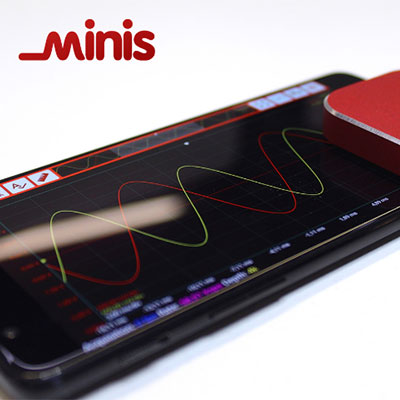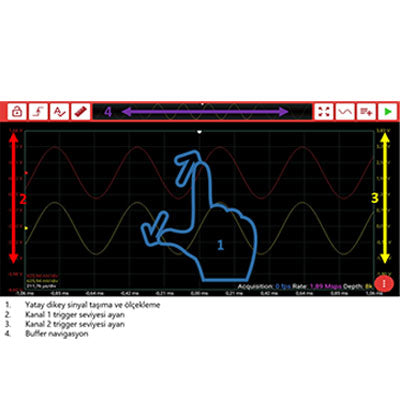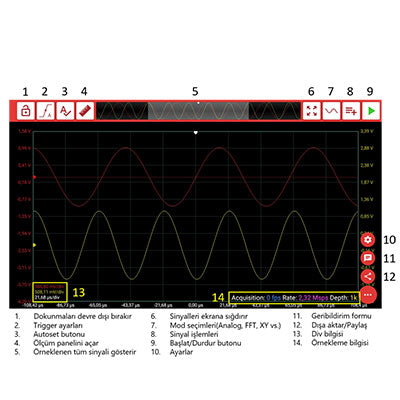#Oscilloscope is an electronic device that allows us to see and calculate the properties of the signals along with their waveforms. The oscilloscope is one of the most used measuring instruments in electronics. Displays the relationship between the two marks applied to the X and Y channels on one screen. In the case that these signs are periodic functions and under special conditions, the figures standing on the screen are obtained. Thus, the time relation between the two functions can be easily monitored on a standing shape. Shortly, the oscilloscope can be defined as an instrument that shows the sudden value of electrical signs and their change over time.
Vertical (y) axis of the image formed on the oscilloscope screen shows the amplitude, that is, the intensity of the measured signal. The horizontal axis shows the time.# How to Use An Oscilloscope

## Probes

A probe is attached to the input signal channel after the oscilloscope is operated. Usually two types of measuring probes are used. These are X1 probes that do not weaken the signal and X10 probes that weaken the signal 10 times. While this second type is working with the probe, if there is a 5 V voltage at the tip of the probe, this voltage reaches the oscilloscope as 0.5 V. If the size of the sign is to be measured, this should be taken into account.
Today, BNC type connectors (plugs) are used in all probes. After these plugs are seated in their places, the moving part on the outside is turned clockwise and locked.
If the sign is to be measured in size, that must be take into consideration

## Voltage Measurement with Oscilloscope

The amplitude of the signal on the screen is measured on the vertical axis. The amplitude is first determined in squares on the screen. Then, the actual value of the voltage is determined by multiplying the value indicated by the signal on the VOLTS / DIV input attenuator commutator and framerate. If a probe with attenuator (X10 or X100) is used, the attenuation coefficient should also be take into consideration. The sensitivity of the oscilloscope is increased by turning the VOLTS / DIV commutator clockwise. Frequency Measurement with Oscilloscope Period is measured in modern oscilloscopes instead of frequency. Period measurements are made on the horizontal axis. The length of one period of the waveform in the X axis direction is determined by counting the squares. Then the period of the signal is determined by multiplying the value indicated by the TIMEBASE commutator and the number of frames. The probe used (X1, X10 or X100) does not affect time measurements.### Period or Frequency Measurement

Period is measured in oscilloscopes instead of frequency. Period measurements are made on the X (horizontal) axis. The length of one period of the waveform in the X axis direction is determined by counting the squares. We get the value of the time / div button by multiplying the number of frames. However, if the probe weakens, the attenuation coefficient is multiplied and taken into account.

### Vertical axis (Voltage axis)

The vertical section of the oscilloscope controls the scale of the tension on the screen. This section, which allows the vertical position and volt / div to be controlled one by one, generally has two buttons. More critical tension per divider button allows adjustment of the vertical scale on the screen. Turning the knob clockwise decreases the scale, and turning it counterclockwise also increases it. A smaller scale means that you “zoom in” more to the waveform. The Position button controls the vertical shift of the waveform on the screen. When the knob is turned clockwise, the wave moves down, and counterclockwise it moves to the screen. The position button can be used to separate part of a waveform from the screen.

### Horizontal axis (Time axis)

The horizontal part of the oscilloscope controls the time scale on the screen.The horizontal axis consists of two buttons: position and time / div. If the time / div button is turned clockwise, the number of seconds represented by each section will decrease. Like the vertical system. A longer time may appear on the screen if it is rotated counterclockwise to increase the time scale. The Position button can adjust the horizontal offset to move the waveform to the left or right of the screen. Using the horizontal system, it is possible to set how many periods of a waveform you want to see. It can be removed, multiple peaks and channels of a signal can be shown.### Functions and Keys of the Oscilloscope

• POWER (On / Off) Button: Provides the task of opening and closing.
• Intens: Adjusts the brightness of the line on the screen.
• Focus: Ensures that the line on the screen is appropriately thin.
• Vertical-Horizontal Position: These buttons change the positions of the scan lines in the horizontal and vertical directions.
• Volt / div Button: Selects the input sensitivity from 5 mV / cm to 20 V / cm.
• Time / div Button: Selects the time between 0.5 ms / cm and 0.2 s / cm.
• Holdoff: Controls the delay time between scans
• Trigger Source:
1. Int (internal triggering): Selects the channel 1 or channel 2 mark displayed on the screen as the trigger mark.
2. Line: Selects the mains frequency as the trigger source.
3. Ext (external triggering): Selects a signal to be applied from a signal to the signal.
• Tilt button: This switch is marked as (+) or (-). These marks determine whether triggering will be done in a positive or negative position.
• Dual Button: It allows Channel 1 and Channel 2 to appear simultaneously.